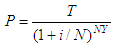# Initial Investments Formula

We're going to implement in Matlab a formula for initial investments.

 This code calculates the amount necessary to provide a stated future value in a specified time period. You must enter the future value of the investment, the number of years, the number of compounding periods per year and the nominal interest rate.

The formula used to calculate initial investments is as follows:where:
P = initial investment
T = future value
N =number of compounding periods per year
i = nominal interest rate

This is our simple Matlab code to calculate the above formula:

function p = initial_investments(t, n, y, it)
it = it/(n*100);
p = t/(1+it)^(n*y);

We create another script to test and run the above m-file. This code starts the previous one:

clc; clear; format bank

t = input('Enter total value after Y years: ');
n = input(
'Enter number of compounding periods per year: ');
y = input(
'Enter number of years: ');
it = input(
'Enter nominal interest rate: ');

init_invest = initial_investments(t, n, y, it)

Example 1:

How much should you invest at 8.5% to produce 10000 at the end of 10 years if interest is compounded quarterly?

Let’s launch our program here...

Enter total value after Y years: 10000
Enter number of compounding periods per year: 4
Enter number of years: 10
Enter nominal interest rate: 8.5

The result is:

init_invest = 4312.38

Example 2:

A savings company wishes to sell a bond which will be worth \$5000 five years from the purchase date. Interest will be 7.9% compounded daily. How much must the bank charge for the bond?

Enter total value after Y years: 5000

Enter number of compounding periods per year: 365
Enter number of years: 5
Enter nominal interest rate: 7.9

The result is:

init_invest = 3368.54

From 'Initial Investments' to home

From 'Initial Investments' to 'Finance Formulas'